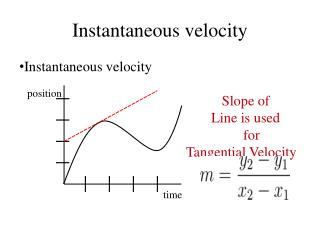# Instantaneous velocity - PowerPoint PPT PresentationDownload PresentationInstantaneous velocity

Instantaneous velocityDownload Presentation## Instantaneous velocity

- - - - - - - - - - - - - - - - - - - - - - - - - - - E N D - - - - - - - - - - - - - - - - - - - - - - - - - - -
##### Presentation Transcript

1. Instantaneous velocity • Instantaneous velocity • Slope of • Line is used for Tangential Velocity position time

2. Graphing Speed: Distance vs. Time Graphs Speed = Slope = Rise/Run Rise

3. Graphing Speed: Distance vs. Time Graphs Speed = Slope = Rise/Run Rise=? 600 km 3 h

4. Graphing Speed: Distance vs. Time Graphs Speed = Slope = Rise/Run Rise=? 600 m 3 minutes Rise/Run = 600 km/3 hr = 200 km/hr

5. Different Slopes Slope = Rise/Run = 0 km/1 hr = 0 km/hr Rise = 2 km Run = 1 hr Rise = 0 km Run = 1 hr Slope = Rise/Run = 2 km/1 hr = 2 km/hr Rise = 1 km Run = 1 hr Slope = Rise/Run = 1 km/1 hr = 1 km/hr

6. Question • Below is a distance vs. time graph of my position during a race. What was my AVERAGE speed for the entire race? Average Speed = Total distance/Total time = 12 km/6 hr = 2 km/hr Rise = 12 km Run = 6 hr

7. Question • Below is a distance vs. time graph for 3 runners. Who is the fastest? Leroy is the fastest. He completed the race in 3 hours

8. Question • What does the slope of a distance vs. time graph show you about the motion of an object? • It tells you the SPEED